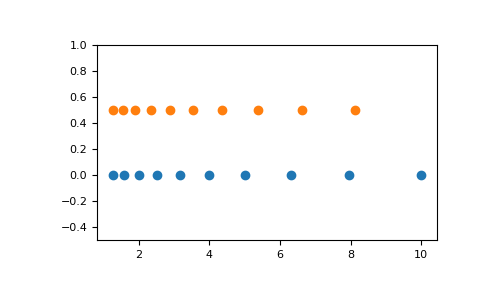# numpy.logspace¶

numpy.logspace(start, stop, num=50, endpoint=True, base=10.0, dtype=None, axis=0)[source]

Return numbers spaced evenly on a log scale.

In linear space, the sequence starts at `base ** start` (base to the power of start) and ends with `base ** stop` (see endpoint below).

Changed in version 1.16.0: Non-scalar start and stop are now supported.

Parameters
startarray_like

`base ** start` is the starting value of the sequence.

stoparray_like

`base ** stop` is the final value of the sequence, unless endpoint is False. In that case, `num + 1` values are spaced over the interval in log-space, of which all but the last (a sequence of length num) are returned.

numinteger, optional

Number of samples to generate. Default is 50.

endpointboolean, optional

If true, stop is the last sample. Otherwise, it is not included. Default is True.

basearray_like, optional

The base of the log space. The step size between the elements in `ln(samples) / ln(base)` (or `log_base(samples)`) is uniform. Default is 10.0.

dtypedtype

The type of the output array. If `dtype` is not given, the data type is inferred from start and stop. The inferred type will never be an integer; `float` is chosen even if the arguments would produce an array of integers.

axisint, optional

The axis in the result to store the samples. Relevant only if start or stop are array-like. By default (0), the samples will be along a new axis inserted at the beginning. Use -1 to get an axis at the end.

New in version 1.16.0.

Returns
samplesndarray

num samples, equally spaced on a log scale.

`arange`

Similar to linspace, with the step size specified instead of the number of samples. Note that, when used with a float endpoint, the endpoint may or may not be included.

`linspace`

Similar to logspace, but with the samples uniformly distributed in linear space, instead of log space.

`geomspace`

Similar to logspace, but with endpoints specified directly.

Notes

Logspace is equivalent to the code

```>>> y = np.linspace(start, stop, num=num, endpoint=endpoint)
...
>>> power(base, y).astype(dtype)
...
```

Examples

```>>> np.logspace(2.0, 3.0, num=4)
array([ 100.        ,  215.443469  ,  464.15888336, 1000.        ])
>>> np.logspace(2.0, 3.0, num=4, endpoint=False)
array([100.        ,  177.827941  ,  316.22776602,  562.34132519])
>>> np.logspace(2.0, 3.0, num=4, base=2.0)
array([4.        ,  5.0396842 ,  6.34960421,  8.        ])
```

Graphical illustration:

```>>> import matplotlib.pyplot as plt
>>> N = 10
>>> x1 = np.logspace(0.1, 1, N, endpoint=True)
>>> x2 = np.logspace(0.1, 1, N, endpoint=False)
>>> y = np.zeros(N)
>>> plt.plot(x1, y, 'o')
[<matplotlib.lines.Line2D object at 0x...>]
>>> plt.plot(x2, y + 0.5, 'o')
[<matplotlib.lines.Line2D object at 0x...>]
>>> plt.ylim([-0.5, 1])
(-0.5, 1)
>>> plt.show()
```